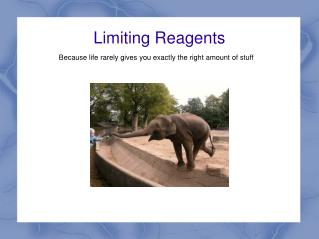DownloadDownload PresentationLimiting Reagents

# Limiting Reagents

Download Presentation## Limiting Reagents

- - - - - - - - - - - - - - - - - - - - - - - - - - - E N D - - - - - - - - - - - - - - - - - - - - - - - - - - -
##### Presentation Transcript

1. Limiting Reagents Because life rarely gives you exactly the right amount of stuff

2. Limiting Reagents At this point, you will have done the S'mores activity in class, hopefully. Given that, you should have some idea that because things can be mixed in whatever proportion you want, but only react in a certain proportion, you will often end up running out of one reactant before the other.

3. Limiting Reagents Therefore, this presentation is just about some techniques you can use.

4. Identifying the Limiting Reagent Let's start with a sample reaction: 2Na3PO4 + 3CaO → Ca3(PO4)2 + 3Na2O • 10 moles 12 moles

5. Identifying the Limiting Reagent Let's start with a sample reaction: 2Na3PO4 + 3CaO → Ca3(PO4)2 + 3Na2O • 10 moles 12 moles Method 1: Just calculate the yield using each compound normally: 10 moles Na3PO4 yields 5 moles Ca3(PO4)2 and 15 moles Na2O 12 moles CaO yields 4 moles Ca3(PO4)2 and 12 moles Na2O

6. Identifying the Limiting Reagent Let's start with a sample reaction: 2Na3PO4 + 3CaO → Ca3(PO4)2 + 3Na2O • 10 moles 12 moles Method 1: Just calculate the yield using each compound normally: 10 moles Na3PO4 yields 5 moles Ca3(PO4)2 and 15 moles Na2O 12 moles CaO yields 4 moles Ca3(PO4)2 and 12 moles Na2O Whichever one gives you less is the one that will run out first (limiting)

7. Identifying the Limiting Reagent Let's start with a sample reaction: 2Na3PO4 + 3CaO → Ca3(PO4)2 + 3Na2O • 10 moles 12 moles Method 1: How much of the Na3PO4 will be used up? Use the ratio 8 moles of Na3PO4 will react with the 12 moles of CaO

8. Identifying the Limiting Reagent Let's start with a sample reaction: 2Na3PO4 + 3CaO → Ca3(PO4)2 + 3Na2O • 10 moles 12 moles Method 2: Figure out how many times you can do the reaction, based on the amount you have, and how many it takes each time. 10 moles Na3PO4 / 2 = enough to do it 5 (moles of) times 12 moles CaO / 3 = enough to do it 4 (moles of) times

9. Identifying the Limiting Reagent Let's start with a sample reaction: 2Na3PO4 + 3CaO → Ca3(PO4)2 + 3Na2O • 10 moles 12 moles Method 2: Take this number of times, and multiply it by the coefficient of anything you're interested in: 4 (moles of) times * 2 = 8 moles Na3PO4 used 4 (moles of) times * 1 = 4 moles of Ca3(PO4)2 4 (moles of) times * 3 = 12 moles of Na2O

10. Either Way We Get This Let's start with a sample reaction: • 2Na3PO4 + 3CaO → Ca3(PO4)2 + 3Na2O Starting amount: 10 moles 12 moles 0 0 Amount Used: 8 moles 12 moles Final Amount: 2 moles 0 4 moles 12 moles

11. Or, In Grams Let's start with a sample reaction: • 2Na3PO4 + 3CaO → Ca3(PO4)2 + 3Na2O Starting amount: 1640g 673g 0 0 Total: 2313g Final Amount: 328g 0 1241g 744g Total: 2313g Conservation of mass still applies, but you get less products out than the mass you put in, because some of it never reacted.

12. Summary • Two methods. Both give the same results. • Everything still reacts in the reaction equation proportions. • You will have less products than what you put in, but conservation of mass still applies—some just isn't used. • S'mores are delicious.